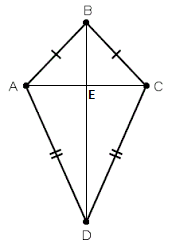Checkout JEE MAINS 2022 Question Paper Analysis : Checkout JEE MAINS 2022 Question Paper Analysis :

# Congruence Of Triangles: RHS And SSS

You are already aware of the term ‘congruency of triangles’. Two triangles are said to be congruent to each other if the measurements of their three sides and their three angles are exactly the same. They may be rotated or flipped. But when you carve them in a piece of paper, cut them out and place one on top of the other, they cover each other perfectly. In this article, we will discuss two important criteria for congruence of trianglesRHS (Right angle – Hypotenuse – Side) and SSS (Side – Side – Side).

## RHS Congruence Rule

Theorem: In two right-angled triangles, if the length of the hypotenuse and one side of one triangle, is equal to the length of the hypotenuse and corresponding side of the other triangle, then the two triangles are congruent.

## SSS Congruence Rule

Theorem: In two triangles, if the three sides of one triangle are equal to the corresponding three sides (SSS) of the other triangle, then the two triangles are congruent.

### Solved Example

Question: In the following figure, AB = BC and AD = CD. Show that BD bisects AC at right angles.Solution: We are required to prove ∠BEA = ∠BEC = 90° and AE = EC.

Consider ∆ABD and ∆CBD,

AB = BC                                                 (Given)

BD = BD                                                (Common)

Therefore, ∆ABD ≅ ∆CBD                 (By SSS congruency)

∠ABD = ∠CBD                                     (By CPCT)

Now, consider ∆ABE and ∆CBE,

AB = BC                                                (Given)

∠ABD = ∠CBD                                     (Proved above)

BE = BE                                                (Common)

Therefore, ∆ABE≅ ∆CBE                  (By SAS congruency)

∠BEA = ∠BEC                                     (CPCTC)

And ∠BEA +∠BEC = 180°                 (Linear pair)

2∠BEA = 180°                                    (∠BEA = ∠BEC)

∠BEA = 180°/2 = 90° = ∠BEC

AE = EC                                                (CPCTC)

Hence, BD is a perpendicular bisector of AC.